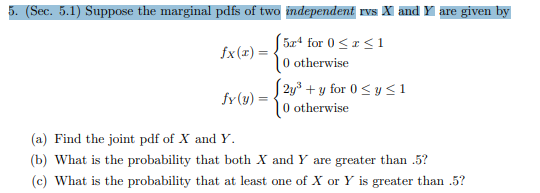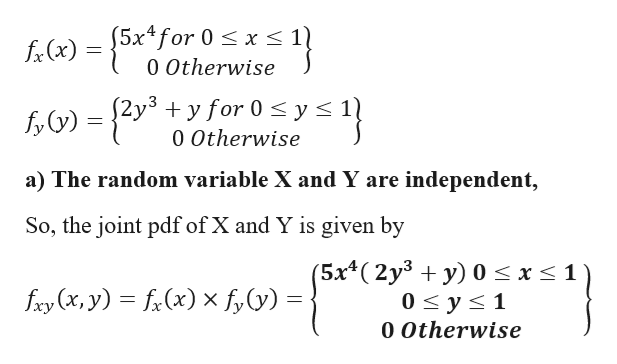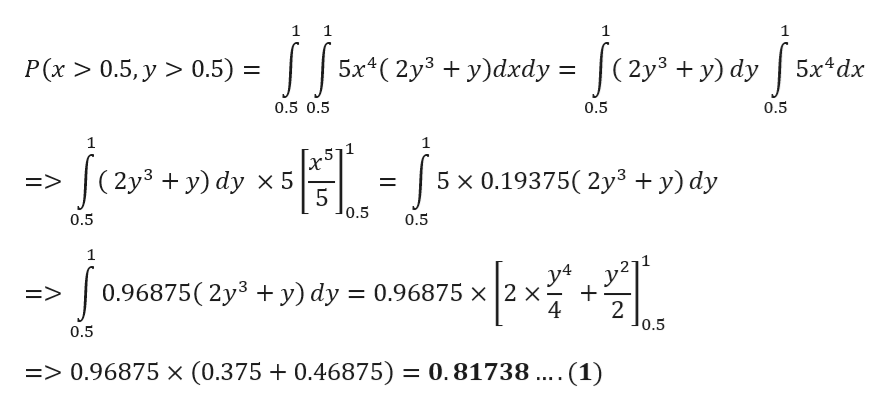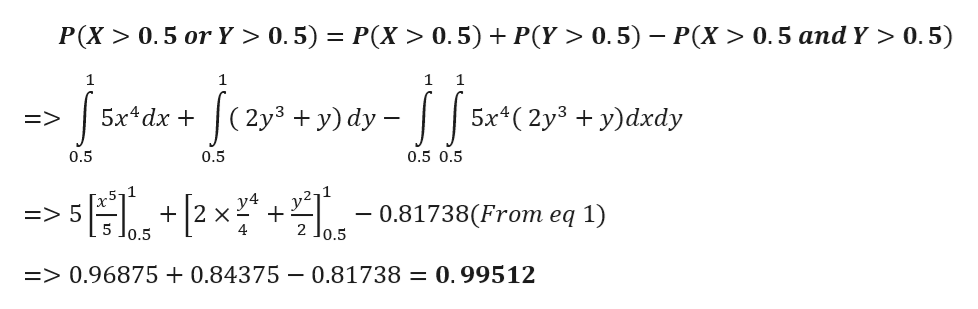# 5. (Sec. 5.1) Suppose the marginal pdfs of two independent rvs X and Y are given by5x4 for 0|0 otherwise1fx(r)2y3for 0y

Question
1 viewshelp_outlineImage Transcriptionclose5. (Sec. 5.1) Suppose the marginal pdfs of two independent rvs X and Y are given by 5x4 for 0 |0 otherwise 1 fx(r) 2y3for 0y <1 |0 otherwise fy(y) (a) Find the joint pdf of X and Y (b) What is the probability that both X and Y are greater than .5? (c) What is the probability that at least one of X or Y is greater than .5? fullscreen
check_circle

Step 1

Given marginal p.d.f of random variable X and Y are given byhelp_outlineImage Transcriptionclose5x4for 0 x s 0 Otherwise fx(x) y for 0 y < fy)=2y +yfor 0s ys 1) 0 Otherwise a) The random variable X and Y are independent, So, the joint pdf of X and Y is given by (5x4(2y3 + y) 0 < x < 1 0 y s 1 fxy (x, y) fi(x)xf,y) = 0 Otherwise fullscreen
Step 2

b)
Probability that both X and Y are greater than 0.5 is given byhelp_outlineImage Transcriptionclose1 1 1 5x(2y3 y)dxdy = (2y3 y) dy P(x >0.5, y > 0.5) = 5x4dx 11 0.5 0.5 0.5 0.5 1 (2y3 y) dy x 5 5 x 0.19375( 2y3 + y) dy => 5 0.5 0.5 0.5 1 y4 0.96875( 2y3 + y) dy 0.96875 x 2 x 4 E> 2 0.5 0.5 => 0.96875 x (0.375 + 0.46875) = 0.81738 .... (1) fullscreen
Step 3

c) Probability that atleast one of X or Y is gre...help_outlineImage TranscriptioncloseP(X> 0.5 or Y > 0.5) P(X > 0.5) P(Y > 0.5) P(X> 0.5 and Y > 0.5) 1 1 1 (2y3 +y) dy 5х4dx + 5x*(2y3 + у)dxdy => 0.5 0.5 0.5 0.5 y4 X 4 0.81738(From eq 1) => 5 5 Jo.5 2 Jo.5 => 0.96875+0.84375 - 0.81738 = 0.99512 fullscreen

### Want to see the full answer?

See Solution

#### Want to see this answer and more?

Solutions are written by subject experts who are available 24/7. Questions are typically answered within 1 hour.*

See Solution
*Response times may vary by subject and question.
Tagged in

### Other